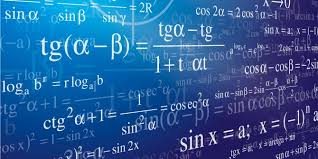Elementary Math

Mathematics is not about numbers, equations, computations or algorithms: it's about understanding

 The Auburn City School District will develop mathematically proficient students through a focus on reasoning and deeper understanding. The district will challenge each student to develop and extend mathematical proficiency through a focused and coherent curriculum that meets the needs of each student with a focus on essential concepts and processes of mathematics. The mathematics curriculum will prepare students to think critically, problem solve, innovate, communicate and collaborate.Links CBT Questions Challenging word Problems Common Core Resources Embarc Fraction Talk Greatminds Parent Support Homework Help K-6 Resource Folders Math Games Math Intervention Websites Numerous Math Games Parent Homework Help (Modules) Question of the Day Standards Assessments Standards test questions
District

Schools